## Thursday, November 8, 2012

### Density Gizmo

This week, with a Density Gizmo in Science, our students continued to deepening their understanding of matter, mass, and volume, and explored density. They first brainstormed objects that they think would sink in water and then those that would float, and formed a hypothesis for why the objects sink or float.

After that, they did a Gizmo warm-up which familiarized them with the virtual exploration by measuring the mass of objects on a scale, and measuring the volume of objects using water displacement in a graduated cylinder.

The essential question then focused their activity, How do mass and volume affect sinking and floating?

During the Gizmo, students filled in a chart with each object's mass and volume, and then whether the object would sink or float when placed in the beaker.  This is a sample of their chart.

 Object Prediction (sink or float?) Mass Volume Result (sink or float?) Ping pong ball F 3.0 g 36.0 mL Float Golf ball S 45.0 g 36.0 mL Sink Apple F 33.0 g 44.0 mL Float Chess piece S 40.0 g 80.0 mL Float Penny S 3.0 g 0.4 mL Sink Rock S 200.0 g 50.0 mL Sink

They analyzed their results and concluded that you could not predict whether an object would sink or float using the mass alone, because the mass of a ping pong ball and penny were both 3 grams and one floated while the other sank. Based on the volume alone, they concluded that you could not predict whether an object would float or sink, because the volume of a ping pong ball and golf ball were both 36 mL and one floated while the other sank.

However, mass and volume, when considered together could predict whether an object would sink or float. When an object's mass was less than the object's volume, the object floated.  When an object's mass was more than an objects volume, then the object would sink. Density refers to the mass found in a given volume of a substance.

These are third graders that I teach and they are taking it in like sponges! They even clapped when we said, "Today, we will be doing a Density Gizmo!" It doesn't get any better than that!

### Decomposing Arrays and Multiples of Ten

Our students' knowledge of multiplication has come so far in so little time. We worked on finding the total number of squares (or area) in an array. An array is a multiplication model used to work toward independence and mental math strategies.

Students discovered that by decomposing the array into smaller arrays, they could more easily find the product. We also encouraged them to record using correct algebraic notation. You'll notice in this piece of student work that 4 x 3, said, "four groups of three," can be decomposed into (2 x 3) + (2x3) = 12.

More recently, we've been exploring larger numbers, too, and recognizing relationships. The chart below was created by my co-teach partner, Ashley, during a lesson to emphasis why students have been seeing the pattern of a 0 in the ones place. Zeros aren't merely added to the ones place, rather they are in the ones place because they are a multiple of 10. Multiples of 10 have a 0 in the ones place. A student could see that 5 x 60 = 5 x 6 x 10. This helps them see why the pattern occurs.

## Monday, October 29, 2012

### How is the Mass and Volume of Matter Measured?

Today our young scientists participated in a science lab to answer the essential question, How can mass and volume be measured?

To get started,  students had to make a hypothesis about which has more mass a crayon or a pencil. Then, using a pan balance with gram weights, they had to measure the mass of each.

After that, they made a hypothesis on whether a marble or a seashell had more mass, and then measured the mass of each. (When the groups analyze their data, they will realize that the mass of all the marbles in the class are the same, however the mass of the seashell changes. The smaller the seashell the less the mass, the bigger the seashell, the larger the mass.)

Furthermore, students made a prediction about the volume of two cups of liquid, and then used a graduated cylinder to measure each volume.  (The red liquid was in a tall narrow glass and the blue liquid was in a small wide glass. Each contained 150 mL of colored water.)

Students were surprised to discover that they could also find the volume of a solid. An object's volume is the amount of space the object takes up. To find the volume of a solid, like the marble and the seashell, students used water displacement. They put 100 mL of water in a graduated cylinder, then gently dropped the solid in the water. The volume is recorded by the number of mL of water that was displaced, or moved.
Tomorrow, we will have Closing Session where students will have to compare and then explain their results. By the end of Science Workshop tomorrow, students will be able to tell how the mass of matter is measured, and how the volume of a solid and liquid is measured.

### Multiplication Strategies

Young mathematicians’ first formal introduction to multiplication and division happens in 3rd Grade.

In our unit, Equal Groups, situations are presented in context. The situation usually requires students to identify the number of groups and the number of items within each group.

There are 5 tricycles. Each tricycle has three wheels. How many wheels are there in all?

Tricycles have three wheels. There are 5 tricycles. How many wheels are there in all?

Number of Groups: 5
Number of Items in Each Group: 3

Students are taught to represent the problem with both an addition equation and a multiplication equation to illustrate the connection, and use a variable for the missing piece of information.

Multiplication equation: 5x3=w (read 5 groups of 3)

Students generally begin solving the multiplication situations with their prior knowledge of repeated addition.

Repeated Addition: 3+3+3=9 and 3+3=6, so 9+6=15.

Then, some students move into skip counting if they can easily skip count by that number. If the situation allows counting skip counting by 2’s, 5’s, and 10’s, students will almost always start with this strategy. If however, the situation has them skip counting by 8’s, then we’ll teach students to use 10 as their anchor. Skip count by 10 and go back 2.

Skip Counting: 3,6,9,12,15.

One tool that we teach students to use to organize their thinking when skip counting is a ratio table. Ratio tables help students keep track of the number of groups as they skip count.During the unit, students are introduced to multiplication situations using arrays, too. An array is a rectangular arrangement with rows and columns.To create a common language with our students, we have them give us the dimensions of the row first and then the column. The array above would be a 5x3.

There are many ways to solve the array. One of the things students do is skip count the array.
They can skip count the array be either the rows or columns.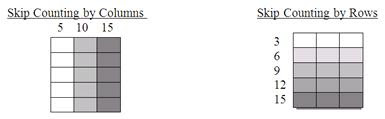Students naturally begin to see and explore the commutative property of multiplication. Though the situation is 5x3, they realize they can applying their number knowledge properties, and solve 3x5 instead (5,10,15 or 5+5+5=15).

Students’ next level of understanding develops when they recognize that they can decompose an array into smaller arrays to help them solve problems.

In this situation, if a student didn’t know the product of 5x3 quickly, they could decompose the array into (5x2) + (5x1) = 15.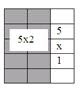This understanding is extremely important as students move into problems that are more difficult when they begin multiplication like 6x8. When in the early stages of developing automaticity, they may not know 6x8, but if they know (3x8), they can solve 2(3x8), or, if they know 6x4, they can solve 2(6x4).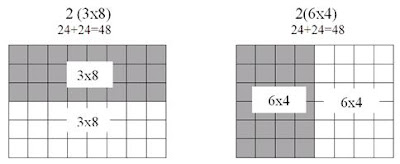Students’ ability to think flexibly with decomposing arrays in multiple ways, builds a strong foundation for fluency in multiplication. The skill allows students to attack any multiplication equation for which they don’t automatically have a product, and leads into being able to solve more difficult equations like 14 x 12.

After the unit, Equal Groups, students have a solid conceptual foundation and can think about multiplication flexibly. But, if we stop there, they may never become fully fluent. We continue to practice fact fluency with our combination club flash cards, by playing multiplication bingo, doing fluency clicker reviews, and doing a timed fluency snapshot several times a week. The fluency snapshots are presented by similar facts. (5’s and 10’s together) (2’s and 4’s and 8’s together) (3’s, 6’s, 9’s, 12’s together) (7’s) and (11’s). Presentation with similar facts promotes the conceptual understanding we build throughout this unit.

Our goal is for every student to leave third grade knowing each of their multiplication facts within three seconds. This foundational knowledge creates automaticity and will help them be successful in fourth grade as they embark on more complex multiplication problems like 49 x 58.

### Decomposing Arrays

In our multiplication unit, students have been using an array model to help them visualize the organization of things in equal rows. We worked on finding the total number of squares (or area) in an array.  The following is the work that students completed during the math workshop.
An array is a model for multiplication (and eventually division too). The goal is for kids to transfer this thinking to multiplication equations using mental math, without an array present. For example, a student might solve 8x6 as (4x6) + (4x6) or (8x3) + (8x3). They will learn to record it as 2(4x6) and 2(8x3).

### Multiple Charts for Multiplication

As we moved further in our multiplication study, we began examining multiples charts. We have highlighted multiples of all numbers 2-12 and have found the patterns that exist between certain charts. For example, we recognized that the multiples of 6 are also multiples of 3 and that there are twice as many multiples of 3 as there are of 6. We also discovered that we skip count by an odd, even, odd pattern. We also discovered relationships between 3s,6s, and 12s.  Furthermore, students pointed out that 100 is not a multiple of 3,6, or 12. In addition, we began answering questions like, How many 3s are in 30? How many 3s are in 60?

We strategically place the students' multiple charts in their packets so they can make connections and find relationships. Below is an example of the work that students completed in class as part of their multiples study.  You will notice that the 2s, 4s, and 8s charts are all on the same page. On the next page are 3,6, and 12. 5s, 10s, and 20s are together, too. In addition, we place a 9s and 11s together and talk about their relationship to the landmark number 10.

## Saturday, October 6, 2012

### Developing a Student's Passion for Science

If you ask me what I'm passionate about, it's teaching students to embrace a love of mathematics, see themselves as young mathematicians, and build a number sense that will allow them to think flexibly about numbers.  Teaching math to children, for me, is second nature.

Science, on the other hand, isn't my forte'. So, I find myself working overtime trying to compensate for what I see as my weaker content area. These kids deserve the the best so though it's not my strength, I must remember it may be their passion. I must, as their teacher, show the same level of competence and enthusiasm for the subject that doesn't come as easily to me. So, as you can imagine, it takes a tremendous amount of planning and preparation, and professional reading on my part to make sure that I offer an engaging science laboratory for my students. I hope that as I'm building young mathematicians, I am also building young scientists.

My goal this year was to plan more hands on experiences. I'm aiming for one a week and so far, I've been pretty successful. The post below is from our lab this week.

### Can You Overcome Gravity?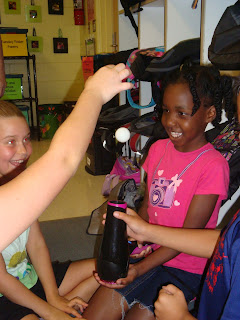Today, our young scientists were busy at work in their laboratory. They were seeking the answer to their essential question, How can the pull of gravity be overcome?  They were given a hairdryer, three ping pong balls, and a cotton ball. Their task was to set the hair dryer on cool, point it toward the ceiling, and then carefully put a ping pong ball in the stream of air.

They concluded that the force of air was stronger than the pull of gravity so the ping pong ball floated in the air stream. Then, they turned the hair dryer slightly to the left and slightly to the right, and noticed that the ping pong ball would follow the air stream and stay suspended, unless, like one group discovered, the stream of air was not strong enough. In that case, the pull of gravity would be stronger and the ping pong ball would be pulled toward Earth.
Next, the young scientists tried floating two or more ping pong balls in the air stream. Some groups were successful while others were not. The discussion behind the mixed results provided an excellent way to introduced the independent variables (What changed?), dependent variables (What did not change?), and constants in this hands on lab.
In addition, the students discovered that floating the cotton ball had a different result. In their exploration, they also discovered that the cotton ball would stick to the bottom of the hairdryer and stop the flow of air.

When this occurred, the forced air would be weaker than the pull of gravity.In our analysis of the lab, students quickly made the connection that the air stream was stronger than the pull of gravity on the mass of the ping pong ball. (Actually, they even strengthened their understanding the following day after a video clip and a reading that the two forces were in balance and that's why the ball levitates in mid-air.)
We asked, "What do you think would happen if we tried the same thing with a golf ball or even a basketball?"  Students concluded that the mass of the golf ball and basketball would be too much for the air stream and gravity would pull it to the ground. However, they also recognized that with a great enough force, they would be able to get the golf ball and basketball to levitate. Some students thought that a leaf blower would work to levitate heavier objects.
In Closing, one student shared that he once was in a machine that made him levitate!  He's bringing a video of the experience in for the class to see. How exciting!

Today's lab explored Bernoulli's Principle, the principle that allows heavier-than-air objects like airplanes to fly. Bernoulli discovered that the faster air flows over an object, the less the air pushes on the surface of the object and so the lower the pressure. In this lab, gravity is pulling on the ball while the pressure from the forced air pushes up on the ball. The forces are balanced,so the ball hovers in the air. You can move the hair dryer from side to side, and the ball will stay hovering in mid-air until one of the forces is stronger than the other.

The students loved the lab and the learning was evident. We hope your young scientist shared their enthusiasm. Furthermore, if you are a student reading this post, we'd love it if you leave us a comment and tell us what you liked most about today's gravity lab.

### Subtraction Strategies

Thinking flexibly about numbers is one of our goals for students in third grade. We’ve been highlighting multiple strategies in Closing Session as we teach students that subtraction is the distance between two numbers. We do this to highlight students' use of an efficient strategies, and as a springboard to challenge students to attempt a strategy that they may not have mastered.  Students quickly realize that some of the strategies are more efficient for particular situations and less prone to computational errors.

Students, as we conclude our subtraction unit,  should be comfortable with multiple subtraction strategies. Solving a problem in using two strategies prevents a computational error that may have been overlooked. Our goal is to have students who can recognize, based on the situation, the most efficient strategy with the least likelihood of error, and with the idea that mental math can be one of the most effective ways to solve.

For example, we don’t want students to use the traditional algorithm to solve an equation like 1000-989=m, because it would be easy for them to make a computational error when regrouping multiple times. Rather, we want students to recognize that the distance between these two numbers can easily be done by counting up, 989+1=990 and 990+10=1000,therefore, the difference is 11.

Of course, in other situations, it's simply easier to solve using the traditional algorithm. In 876-563, the quickest way to the difference is simply using the traditional algorithm.

In order to develop number flexibility, we’ve been working on several strategies in class.
Sample Problem:  245 - 178 = m
Turn the equation into a missing addend 178 + m = 245. Put the number 178 on a number line and count up to the next landmark number. (Landmark numbers have a O or 5 in the ones place.) 178 count up 2 to 180, count up 20 to 200, and then jump 45 from 200 to 245. Adding the jumps gives you the answer, m = 67.

Decomposing
Decompose the number by place value, then subtract each place value. In this problem, 200-100 = 100, 40-70 = -30, 5-8 = -3, therefore 100-30-3= 67.  Sometimes, this strategy has you in negative numbers, but students know that 0 is the middle of the number system and can flexibly use negative numbers. Some students use this strategy and regroup from the larger place value. If they did that in this problem, they would take a group of 100 from 200 and put 140 in the tens place.

245  :     200    40    5
-178  :    -100  +   70  +   8
100   - 30  - 3 = 67

Counting backward
245 - 178 = m

Put 245 on the open number line and count backward 178. You can make the jump of 178 any way you want. Most kids jump backward to landmark numbers. 245 jump back 45 is 100, and then jump back 30 is 70, then jump back 3 is 67. This strategy works best for students who can readily count backward, other students find it difficult.
Left to Right
Students think 200 – 100 = 100 and 40 – 70 = -30 and 5 – 8 = -3.  Then, 100-30 is 70, and 70-3 is 67.
245  :
-178  :
100 – 30 – 3
70 – 3 = 67

Compensation / Creating an Equivalent Problem
In some situations, we also encourage students to compensate to create an easier equivalent problem. Generally, in subtraction, when you create an equivalent problem your goal is to create a problem where regrouping is not necessary. Many students try to create the digit 9 in their minuend.

For example,
56   + 3        59
-47     -3       -44
15

Remember, the purpose of exposing students to multiple strategies is two-fold. First, students need to be able to solve using two different strategies to check their work, and secondly students will be able to identify the strategy that is most efficient based on the problem. Students who successfully accomplish this have number sense and are able to work with numbers mentally and flexibly. Our students are busy every day becoming young mathematicians.

### Math Portfolio Piece, Addition Strategies

From time to time, we have students complete math portfolio pieces to show evidence of students' understanding of a concept or skill. Recently, students completed an addition strategy piece.

The portfolio piece had two problems, 298+574, and a word problem, Mrs. Shall has 321 shells in her seashell collection. Miss Russell has 524 shells in her seashell collection. How many seashells do Mrs. Shall and Miss Russell have if they combine their collections?

Students used two different strategies to solve. Research shows that when students make a computational error and use the same strategy the second time they solve, they commonly make the same mistake. In addition, students with a tool box of strategies are better able to approach each problem and use the strategy that is most efficient for the given problem.

In some situations, the most efficient strategy is the traditional algorithm, but in others, to use more mental math, it may be compensation or left to right addition.

This is a student's sample from our Addition Portfolio Piece. You'll notice that the student used two different strategies to solve 298+574, decomposing by place value and left to right addition.

Decomposing By Place Value
298+574
(200+500) + (90+70) + (8+4)
700        +    160      +   12     =   872

Decomposing by place value is a strategy used by many mathematicians for mental math. The strategy keeps the place value of the numbers, and gives students the opportunity to solve for partial sums by place value.  The strategy, in this situation, avoided the traditional regrouping between place values. You can see the student's understanding of correct algebraic notation, too.

298
+574
700
+  160
12
872

Like decomposing, left to right is a strategy that lends itself to mental math, and keeps the place value of numbers in perspective. You'll notice the student's partial sums are recorded by place value, too.  Again, the student has avoided the traditional strategy of regrouping that the traditional algorithm demands in this equation.

Compensation / Creating an Equivalent Problem
In the second problem, the student also shows his command of at third strategy, compensation. He solves 321+524 by creating an equivalent problem, 321+524 = 330+ 515.

321  (+9)   330
+ 524  (-9)    515
845

When compensating, students create an equivalent problem using landmark numbers. Students can easily solve 330+515 without pencil and paper.

Many of the students on this portfolio piece used compensating for the first problem, too, 298+574.   Compensating to create the equivalent problem 300+572 makes solving the problem so much easier. This type of flexibility in thinking is exactly what adults with good number sense do on a daily basis.

298  (+2)  300
+ 574   (-2)   572
872

We don't avoid the traditional algorithm, but we do insist that students correctly explain it when they solve. In the second problem many students used the traditional algorithm.   This strategy was very efficient because it did not require any regrouping.
321
+  524
845

A few students used the traditional algorithm for the first problem which did require regrouping.
11
298
+574
872

When using this strategy students should be able to explain, "Eight plus four equals 12, I regroup 10 ones and create another group of ten. One group of ten, plus 9 groups of ten, plus seven groups of ten equals 18 groups of ten. I make one group of 100 out of 10 groups of 10.  One group of 100, plus 2 groups of 100, plus 5 groups of 100, equals 8 groups of 100. My sum is 872."

Students reach this level of abstract math understanding by first exploring other strategies. One of the earliest strategies students explore is the open number line.

Open Number Line

The open number line is a concrete strategy that third grade students commonly revert to, particularly when they get stuck, or have conflicting sums in two different strategies.   When using the open number line, we encourage students to start with the largest addend and then add on.  We also encourage them to make the fewest jumps possible. One way of jumping on the open number line is provided in this example.

Regardless of a student's strategy, we are working toward efficiency, flexibility, and good number sense. We know that exposing them to many strategies will assist them in reaching this goal.

## Friday, August 24, 2012

### Organizing Our Anchor Charts

We create Anchor Charts of student learning frequently in our classroom, particularly in our Closing Sessions. Sometimes these anchor charts are a "sloppy copy" completed during the Workshop and meant only to post in the classroom for a short period of time.

However, on other occasions, we spend time after we've created a Closing Session "sloppy copy" with the students to make a polished chart that will hang for the entire unit, because it's a chart that students will have to reference often.  You can see one of those charts, Strategies for Comparing Fractions, hanging on our Math Anchor Chart wall.  I want to reiterate that these charts are never hung until after the Workshop and the "sloppy copy" is made with students.  Some of the charts, that take extra time to create, we laminate and use from year to year.

This year, we decided to be more organized with our charts. A colleague shared her strategy and we loved it. We purchased some pant hangers from Target that have an add on  loop at the bottom of each hanger. The hangers hold a chart and you can add the next hanger on the add on loop. The beauty of the system comes from the easily made stacks and we LOVE the fact that we can see the title of each chart. We  took it one step further and hung the charts by subject and unit for easy accessibility. The display you see below hangs neatly in our closet. We hope you love the idea as much as we do!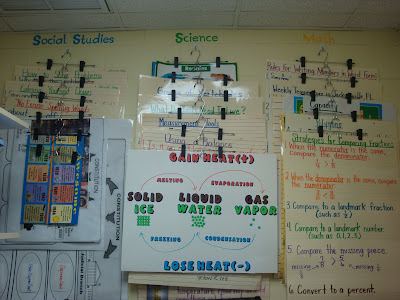### Classroom Centers to Roll Out Rituals and Routines

Like all teachers, my co-teacher and I spent time preparing and organizing our classroom for the arrival of new students this school year. We set the room up strategically as we thought through our systems. Making sure the learning community was set up to promote the growth of independent self-directed learners was our priority. Explaining these systems to students and our expectations was necessary but we wanted to strike the perfect balance between telling and allowing them to explore during those first few days of school.

We decided to cover a few of the essentials that students always ask like,  When are we going to lunch? When is recess? Where is the bathroom? Beyond that, we decided to let the students do some exploring. Nine stations were set up throughout the classroom to cover and explain elements like 1) Lost and Found & PTA Bins   2) Recess materials   3) Math Games and Manipulatives   4) Science Jobs   5) Tray Material Retrieval   6) Materials Bins   7) Class Bank and Store   8)  Research Library   9) Laptops and CPS Clickers. An explanation was hung with each station number and students in groups of three to four visited each station.

Embedded within the explanation were questions for the students to answer, and a few of the stations had a couple fun activities like voting on a name for our classroom fish and guessing the number of cookies in a jar.

We broke the stations into two different segments during the day, so it wasn't too much reading for the students, and when we finished, we quizzed the kids on the material and handed out skittles for correct answers. It really was a great way to cover some of our rituals and routines without having to bore the kids with too much talking. We will certainly do it again next year, and might even add a student recording sheet to help with student participation and accountability.